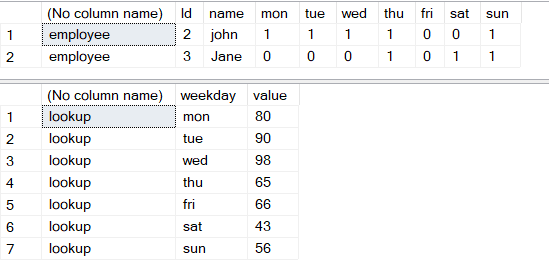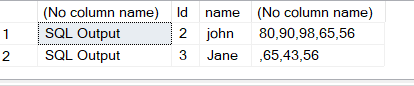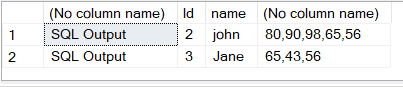# Merge multiple columns into one column separated by a comma

I have a table that has multiple columns and I want them in a single column .however only the values = 1 should be returned

Employee table
Id,name,mon,tue,wed,thurs,fri,sat,sum
2,john,1,1,1,1,0,0,1
3,Jane,0,0,0,1,0,1,1

Output

Id,name,week
2,john,1,1,1,1,1 ——-the 0s are removed
3,Jane,1,1,1

Now to identify each day I have a lookup table
Mon=80
Tue=90
Wed=98
Thu=65
Fri=66
Sat=43
Sun=56

Expected output

Id,name,week
2,john,80,90,98,65 ——-the 0s are removed
3,Jane,65,43,56

do
concat

with
where clause in which values 1 selected

Why would you remove the zeroes?? Now you don't know which columns belong to which day

hi

its easier to write a cursor and loop through ... !!
there are also other ways to do this !!

i tried to do this ONE WAY .. please take a look ..

i hard coded the commas part .. that needs to be fixed ..

please click arrow to the left for create data script
``````drop table #employee

create table #employee
(Id int,name varchar(20),mon int,tue int,wed int,thu int,fri int,sat int,sun int)

insert into #employee select 2,'john',1,1,1,1,0,0,1
insert into #employee select 3,'Jane',0,0,0,1,0,1,1

select * from #employee

drop table #lookup

create table #lookup
(
weekday varchar(20),
value varchar(10)
)

insert into #lookup select 'mon','80'
insert into #lookup select 'tue','90'
insert into #lookup select 'wed','98'
insert into #lookup select 'thu','65'
insert into #lookup select 'fri','66'
insert into #lookup select 'sat','43'
insert into #lookup select 'sun','56'
````````````; with cte as
(
select Id,name
, case when mon =1 then 'mon' else '' end
+','+ case when tue  =1 then 'tue' else '' end
+','+ case when wed  =1 then 'wed' else '' end
+','+ case when thu  =1 then 'thu' else '' end
+','+ case when fri  =1 then 'fri' else '' end
+','+ case when sat  =1 then 'sat' else '' end
+','+ case when sun  =1 then 'sun' else '' end as ok
from #employee
) ,cte_lookup as
(
select
'mon' as mon,max(case when weekday = 'mon' then value end ) as okmon
, 'tue' as tue,max(case when weekday = 'tue' then value end ) as oktue
, 'wed' as wed,max(case when weekday = 'wed' then value end ) as okwed
, 'thu' as thu,max(case when weekday = 'thu' then value end ) as okthu
, 'fri' as fri,max(case when weekday = 'fri' then value end ) as okfri
, 'sat' as sat,max(case when weekday = 'sat' then value end ) as oksat
, 'sun' as sun,max(case when weekday = 'sun' then value end ) as oksun
from #lookup
)
select a.Id,a.name,
replace(replace(replace(replace(replace(replace(replace(replace(replace(a.ok,b.mon,b.okmon),b.tue,b.oktue),b.wed,b.okwed),b.thu,b.okthu),b.fri,b.okfri),b.sat,b.oksat),b.sun,b.oksun),',,',','),',,',',')
from cte a ,cte_lookup b
``````Ok will try this thanks

Hi

I have an idea for the commas

+','+ case when tue =1 then 'tue' else '' end

Do it like this
case when tue =1 then 'tue,' else '' end

For mon to sun

hi

i have fixed the issue of commas ..

``````; with cte as
(
select Id,name
, case when mon =1 then ',mon' else '' end
+ case when tue  =1 then ',tue' else '' end
+ case when wed  =1 then ',wed' else '' end
+ case when thu  =1 then ',thu' else '' end
+ case when fri  =1 then ',fri' else '' end
+ case when sat  =1 then ',sat' else '' end
+ case when sun  =1 then ',sun' else '' end as ok
from #employee
) ,cte_lookup as
(
select
'mon' as mon,max(case when weekday = 'mon' then value end ) as okmon
, 'tue' as tue,max(case when weekday = 'tue' then value end ) as oktue
, 'wed' as wed,max(case when weekday = 'wed' then value end ) as okwed
, 'thu' as thu,max(case when weekday = 'thu' then value end ) as okthu
, 'fri' as fri,max(case when weekday = 'fri' then value end ) as okfri
, 'sat' as sat,max(case when weekday = 'sat' then value end ) as oksat
, 'sun' as sun,max(case when weekday = 'sun' then value end ) as oksun
from #lookup
)
select a.Id,a.name,
STUFF(replace(replace(replace(replace(replace(replace(replace(a.ok,b.mon,b.okmon),b.tue,b.oktue),b.wed,b.okwed),b.thu,b.okthu),b.fri,b.okfri),b.sat,b.oksat),b.sun,b.oksun),1,1,'')
from cte a ,cte_lookup b
````````````;WITH weekday_codes AS (
SELECT '80909865664356' AS weekday_codes
)
SELECT e.Id, e.name, STUFF(
CASE WHEN e.mon > 0 THEN ',' + SUBSTRING(wc.weekday_codes, 1, 2) ELSE '' END +
CASE WHEN e.tue > 0 THEN ',' + SUBSTRING(wc.weekday_codes, 3, 2) ELSE '' END +
CASE WHEN e.wed > 0 THEN ',' + SUBSTRING(wc.weekday_codes, 5, 2) ELSE '' END +
CASE WHEN e.thu > 0 THEN ',' + SUBSTRING(wc.weekday_codes, 7, 2) ELSE '' END +
CASE WHEN e.fri > 0 THEN ',' + SUBSTRING(wc.weekday_codes, 9, 2) ELSE '' END +
CASE WHEN e.sat > 0 THEN ',' + SUBSTRING(wc.weekday_codes,11, 2) ELSE '' END +
CASE WHEN e.sun > 0 THEN ',' + SUBSTRING(wc.weekday_codes,13, 2) ELSE '' END
, 1, 1, '') AS week
FROM #employee e
CROSS JOIN weekday_codes wc``````

This solution works thx a lot